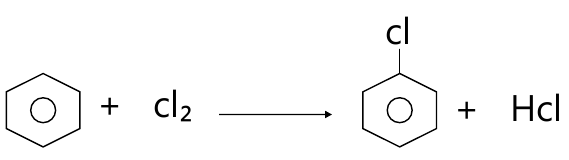MORE IN Applied Chemistry 2
MU First Year Engineering (Semester 2)
Applied Chemistry 2
May 2013
Total marks: --
Total time: --
INSTRUCTIONS
(1) Assume appropriate data and state your reasons
(2) Marks are given to the right of every question
(3) Draw neat diagrams wherever necessary

Answer any five from the following:-
1 (a) Why silver, gold and platinum do not undergo oxidation corrosion?
3 M
1 (b) Define Octane number and Cetane number. Give their significance.
3 M
1 (c) Give the composition, properties and uses of German Silver.
3 M
1 (d) Give classification of composite material.
3 M
1 (e) What is green chemistry? List the 12 principles of green chemistry.
3 M
1 (f) State the characteristics of good paint.
3 M
1 (g) A coal sample was subjected to ultimate analysis. 0.6gm of coal on combustion in a bomb calorimeter, produces 0.05gm of BaSO4. Calculate the percentage of S in the coal sample.
3 M

2 (a) What are metallic coatings? Distinguish between galvanizing and tinning.
6 M
2 (b) Explain refining of petroleum with suitable diagram.
5 M
2 (c) Calculate percent atom economy for following reactions: (w.r.t chlorobenzene).4 M

3 (a) A coal sample has the following composition by weights: C = 82%, H = 3%, O = 8%, S = 2%, N = 2% and Ash = 3%. Calculate the minimum amount of air required both by weight and volume for complete combustion of 2 Kg of coal. (molecular wt. of air = 28.949 gm)
6 M
3 (b) Explain traditional and greener route of production of indigo dye. By this reactions which principle of green chemistry is shown?
5 M
3 (c) How is the rate of corrosion influenced by :-
i) pH of the medium
ii) Relative area of cathode and anode part?
4 M

4 (a) Write a note on compacting and sintering.
6 M
4 (b) Explain wet corrosion in acidic medium with schematic diagram and mechanism.
5 M
4 (c) Explain laminar composite with suitable example.
4 M

5 (a) What is biodiesel? Explain the method to obtain biodiesel from vegetable oil. Give advantages of biodiesel as fuel.
6 M
5 (b) Distinguish between brass and bronze.
5 M
5 (c) State the chemical factors influencing adhesive action.
4 M

6 (a) What is cathodic protection? Describe impressed current method of corrosion control.
5 M
6 (b) A gaseous fuel has the following composition by volume: H2 = 10%, CH4 = 30%, C3H8 = 20%, CO = 20%, CO2= 15%, N2 = 5%. Calculate the volume of air required for complete combustion of 1 m3 of fuel.
5 M
6 (c) Explain the effect of following elements on alloying:-
(i) Nickel
(ii) Chromium
(iii)Cobalt
(iv)Molybdenum
(v)Tungsten
6 M

More question papers from Applied Chemistry 2